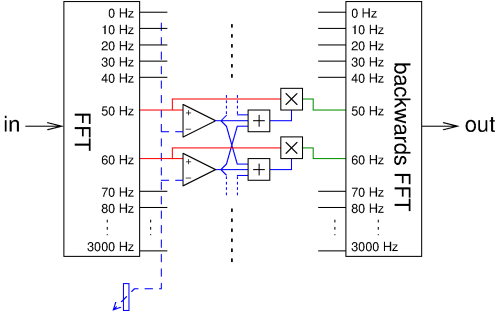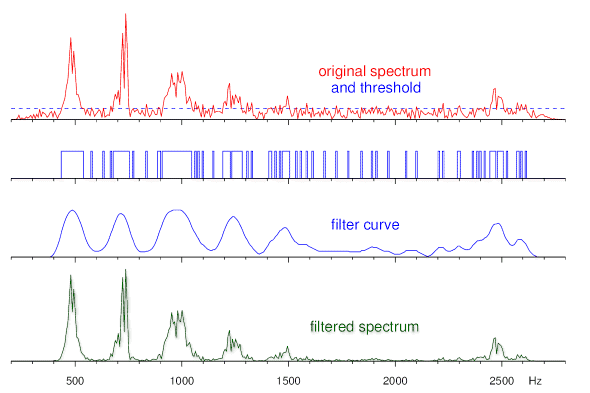# Digital noise reduction

Pieter-Tjerk de Boer, PA3FWM pa3fwm@amsat.org

(This is an adapted version of part of an article I wrote for the Dutch amateur radio magazine Electron, April 2017.)

Since last December, the Twente WebSDR has a user-controllable function for reducing background noise, of which the principle can be explained relatively easily.Consider the figure. We see signals entering at the left and being converted to the frequency domain using an FFT (Fast Fourier Transform). Each output of the FFT thus corresponds to a frequency, or rather, a narrow band of frequencies, here taken in 10 Hz steps for simplicity. So there is an output telling how much 0 Hz (DC) there is in the input signal, one telling how much 10 Hz (and nearby frequencies) there is and with what phase, one for 20 Hz, and so on. Each of these outputs is often called a "bin", based on the idea that signals in a certain frequency range are thrown into that bin. Next, each of these bins is compared to a user-controllable threshold (the variable resistor at the bottom). If the level of the bin is below the threshold, then we open the switch, setting the bin to 0; if it is above the threshold, we pass the bin without any change. The result of this is converted back to the time domain using another FFT. The idea is that bins containing useful audio have a higher level than those containing just background noise, so if we set the threshold correctly, we get rid of the contribution of the bins which contain only noise.

Unfortunately, the above is a bit too simple. There are two reasons for this. The first reason is that noise is a random process, and the level in the noise bins therefore fluctuates. As a consequence, even a bin containing only noise will occasionally be above the threshold, and become audible. This "flapping" does not sound nice.

The second reason is the fact that bins are not sharply delineated in frequency. A tone of e.g. 63 Hz will not only land in the nearest bin, in this case the one for 60 Hz, but also in the 70 Hz bin, and also in other nearby bins. If some of these bins are set to 0 and others are not, that tone will be distorted in very strange way (e.g., rattling at the rate at which the FFTs are calculated). One can filter in the frequency domain between the forward and backward FFT, but it must be done very carefully; just setting a few bins to 0 is not allowed, and leads to distortion. What is allowed, is multiplying all bins by coefficients which have been calculated precisely using a bunch of mathematics; under some conditions one then gets a filter that does not give any distortion.Both problems can be solved as sketched at the right. We still check for every bin whether it is above or below the threshold. However, we no longer switch the bins purely on and off. Instead, we multiply each bin by a number between 0 and 1, which is determined by several nearby bins: the more of those bins are below the threshold, the lower the multiplication factor. For example, one could consider the bin itself and its four neighbours on either side, count how many are above the threshold, and divide that by nine. If all nine bins are above the threshold, the result is 1 and the bin is passed unchanged. If they are all below the threshold, it results in 0 and thus the bin is set to 0. And other cases result in something in between, attenuating the bin. (For simplicity, in the figure only one bin on either side is taken into account, not four.)The next figure shows this algorithm in action on real signals. At the top, we see what comes out of the first FFT: at this moment, apparently there are some peaks in the audio spectrum near 500, 700, 1000, 1200 and 1500 Hz, while the rest seems noise. The dotted line represents the (user-controllable) threshold. The first blue plot shows which bins are above and below the threshold. The second blue plot shows the "smoothed" version of this, i.e., the result of also taking neighbouring bins into account; this curve is the filter which will be applied to the input signal. The bottom plot shows the result: the peaks in the spectrum have hardly changed, but the noise inbetween has been significantly reduced. Be aware that all of this is repeated about 10 times per second, every time processing a fresh piece of input signal; thus, the peaks of the filter curve follow the peaks in the spectrum.

How does this "smearing out" of the threshold values solve the problems mentioned before? The first problem (the "flapping") is solved by the fact that a decision about a bin now no longer depends on only that bin itself, but also on its neighbours. So even if the bin itself occasionally happens to be above the threshold, its neighbours most likely are not, so it will still be multiplied by a number that is close to 0, setting the bin to almost 0. In the example we see this clearly around 2000 Hz. The second problem (the distortion) is solved by the fact that the multiplication factors for adjacent bins do not differ much (because almost the same set of bins contribute to their calculation); as a consequency, in case of e.g. a 63 Hz tone, it can no longer happen that the 60 Hz bin is passed unchanged while the 70 Hz bin is set all the way to 0. Mathematically this still isn't quite right, but we get away with it because these are bins which contain only a small part of the total signal power (after all, most power is in the bins that we do not want to attenuate), and a bit more distortion in that little bit of signal goes unnoticed.

In many situations this algorithm works surprisingly well. E.g., on a weak mediumwave broadcast station it can improve reception enough to make music enjoyable. However, setting the threshold is rather critical. For the weakest signals, where one has most need for noise reduction, the algorithm unfortunately doesn't work well: the bins with useful signal do not stand out enough above the only-noise bins to reliably discern them.

B.t.w., I did not invent this algorithm myself. WebSDR users pointed out that the SDR# software features good digital noise reduction. Since the source code of SDR# is publicly available, I could have a look to see what algorithm they use, and implement something similar in the WebSDR, albeit adapted to efficiently handle hundreds of simultaneous users. Unfortunately it didn't say where the SDR# developers got the idea for this algorithm, or whether they invented it themselves. In any case, it looks quite like the classical "spectral subtraction" algorithm from scientific literature .

## References:

 Steven F. Boll: Suppression of acoustic noise in speech using spectral subtraction. IEEE Tr. on acoustics, speech, and signal processing, 1979. (online at IEEE)

Text and pictures on this page are copyright 2017, P.T. de Boer, pa3fwm@amsat.org .
Republication is only allowed with my explicit permission.